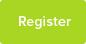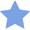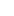# Tutor Hunt Questions

A trolley of mass 0.20kg travelling at 1.5 m/s to the right collides with a trolley of mass 0.30kg moving in the opposite direction at 0.5 m/s.

A) Calculate the total momentum before the collision.

B) Calculate the velocity of the trolleys after the impact if they move off together.

I know the answers but need an explanation on how to work this out properly.
3 years ago

) Calculate the momentum for each trolley separately. This is simple enough, as you are given the individual mass and velocity of each trolley. Now, this is the difficult part: momentum is a vector quantity, meaning it has both magnitude and direction. This means you will have to choose one direction (ie direction of A or B) to be `positive`, and one to be negative. You can think of this as being because the trolley travelling in the opposite (or negative) direction cancels out some of the momentum of the `positive` direction trolley. Which direction is positive is up to you - I advise choosing the direction with greater momentum. For the total momentum, simply add the momentum of one trolley (which will be a positive number) to that of the other (which will be negative).
22/02/2016 18:23:50 | comment by Akanksha
Momentum is the product of mass and velocity, or `p=mv`. A) The TOTAL momentum inside this system is both the momentum of the first trolley and the second trolley individually before they collide. 1st trolley, p=mv, p=(0.20kg)*(1.5m/s)=0.30kgm/s 2nd trolley, p=mv, p=(0.30kg)*(0.5m/s)=0.15kgm/s So the total momentum is 0.30 + 0.15 =0.45kgm/s. B)If they move off together, then their masses will combine, giving us the `m` part of the p=mv formula. (0.20kg + 0.30kg)=0.50kg. The law of conservation of momentum says that the total momentum before a collision = the total momentum after a collision. As such, we will use `p` as the value you just calculated in part A. If we rearrange p=mv, we find that v=p/m. Plug in the values: v=p/m, =0.45/0.50= 0.9m/s Hope this helped :D Source- A level student
22/02/2016 19:42:51 | comment by James

Please enter your response to the question below. The student will get a notification as soon your response has been approved by our moderation team.

####Accepted Answer

The point of this exercise is to employ the notion of conservation of momentum. The momentum of each trolly can be calculated using p = mv. This yields p = 0.3 Kgm/s for the first trolly and p = -0.15 Kgm/s for the second. Notice the minus sign, this is because the two trollies travel in opposite direction.

The total momentum is simply the sum of these two results, p_tot = 0.3 - 0.15 = 0.15 Kgm/s.

Part (B) can be answered thanks to the fact that momentum is conserved, ie. the total is the same before and after the collision:
p = 0.15 = mv = (0.2+0.3)v = 0.5v
v = 0.15/0.5 = 0.3 m/s
2) by the principle of conservation of momentum, the momentum before (ie sum of the individual momenta) equals the total momentum of the two trolleys (now moving as one, and can therefore be treated as one object)

Using the equation momentum (p) = mass (m) times velocity (v), you can take the momentum you worked out in (1) and rearrange the equation to work out the velocity, where the mass of the object is simply the sum of the mass of the two trolleys.

I hope this has made things clearer.
Answered by Akanksha | 3 years ago
It works on a simple law. conservation of momentum.

Conservation of momentum is, Momentum before collision = Momentum after collision.

M1U1+M2U2=M1V1+M2V2

A) M1= 0.2Kg, U1=1.5m/s M2=0.3Kg, U2=-0.5m/s(Velocity is a vector and it has got direction and magnitude).

Momentum before collision = M1U!+M2U2=0.2*1.5+0.3*(-0.5)=0.3-0.15=0.15Kg-m/s

B) If they move together, then both the bodies have got the common velocity

Momentum after collision = M1V1+M2V2= (M1+M2)*V2=(0.2+0.3)*V2=0.5V2 Kg-m/s

Momentum before collision = Momentum after collision
0.15Kg-m/s = 0.5V2
V2=0.3m/s.
Both the bodies after collision move with a velocity 0.3m/s towards right direction.